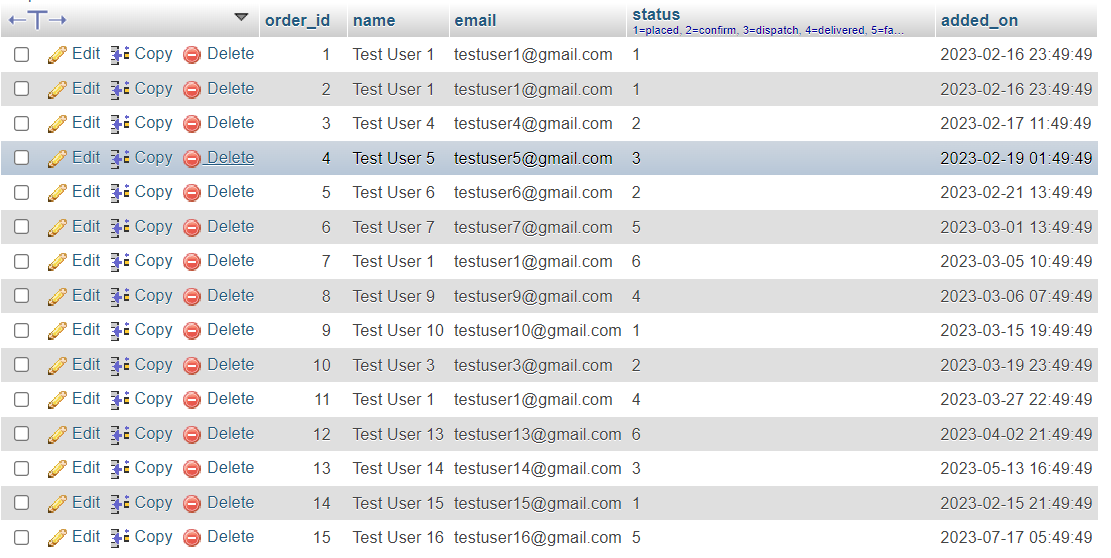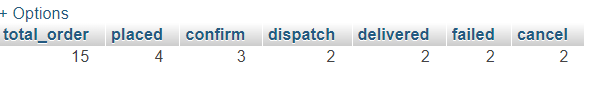# SQL - Total Orders Count According to Status## SQL - Total Orders Count According to Status

##### Published Feb 16,2023 by Kailash Singh

0 Comment     81 Views

In this tutorial, we are going to teach you, how to show order count according to status using SQL.

Example: You have a table of order records. Inside the table you have different types of status records.

LIKE: placed, confirm, dispatch, delivered, failed and cancel.

Table view:SQL Query:

``````SELECT
count(order_id) as total_order,
sum(if(status=1,1,0)) as placed,
sum(if(status=2,1,0)) as confirm,
sum(if(status=3,1,0)) as dispatch,
sum(if(status=4,1,0)) as delivered,
sum(if(status=5,1,0)) as failed,
sum(if(status=6,1,0)) as cancel
FROM
`orders`;``````

Result: